## Split text string at specific character in a cell in Excel

This tutorial explains how to split texts string at a specific character into separate column cells with formulas in Excel.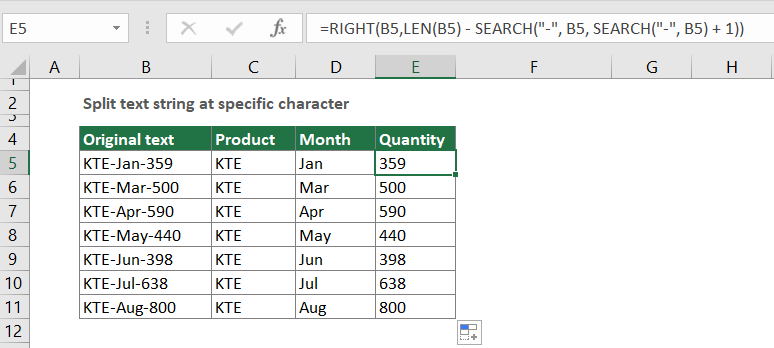#### How to split text string at specific character in a cell in Excel?

Supposing you have a text string list in column B as the below screenshot, and you want to split texts at the character “-” and output them in three separate columns. In this case, the below formulas can do you a favor.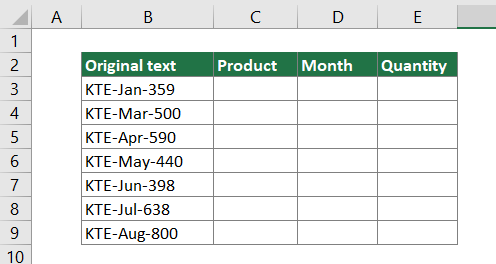Generic formulas

Generic formula for splitting the left text

=LEFT(text_string,FIND("Char", text_string)-1)

Generic formula for splitting the middle text

=MID(text_string, FIND("Char", text_string) + 1, FIND("Char", text_string,FIND("Char", text_string)+1) - FIND("Char", text_string) - 1)

Generic formula for splitting the right text

=RIGHT(text_string,LEN(text_string) - FIND("Char", text_string, FIND("Char", text_string) + 1))

Arguments of above formulas

Text_string: The text string that you want to split text from;

Char: The character you will split text based on.

How to use these formulas?

##### Firstly, split the first text string based on specific character

1. Select a blank cell to output the result. Here I select C3.

2. Enter the below formula into it and press the Enter key. And then drag the cell with your LEFT formula all the way down to apply it to other cells.

=LEFT(B3,FIND("-",B3)-1)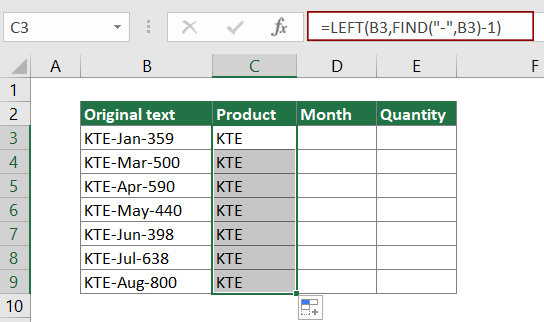##### Secondly, split the middle text string based on specific character

1. Select a blank cell such as D3 to output the result.

2. Enter the below formula into it and press the Enter key. Select the result cell, drag the Fill Handle down to apply the formula to other cells.

=MID(B3, FIND("-",B3) + 1, FIND("-",B3,FIND("-",B3)+1) - FIND("-",B3) - 1)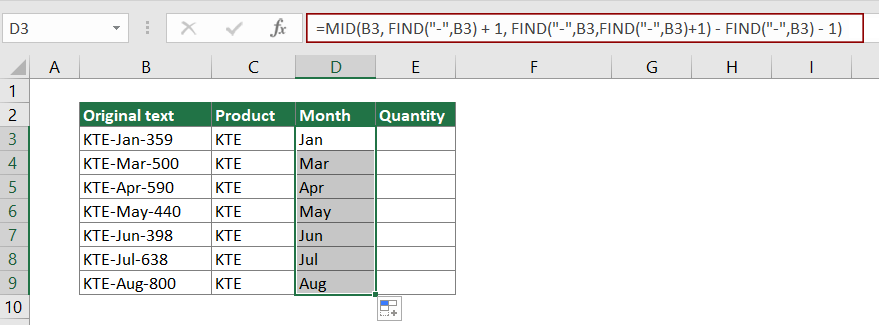##### Finally, split the last text string based on specific character

1. Select a blank cell such as E3 to output the result.

2. Enter the below formula into it and press the Enter key. Select the result cell, drag the Fill Handle down to apply the formula to other cells.

=RIGHT(B3,LEN(B3) - FIND("-", B3, FIND("-", B3) + 1))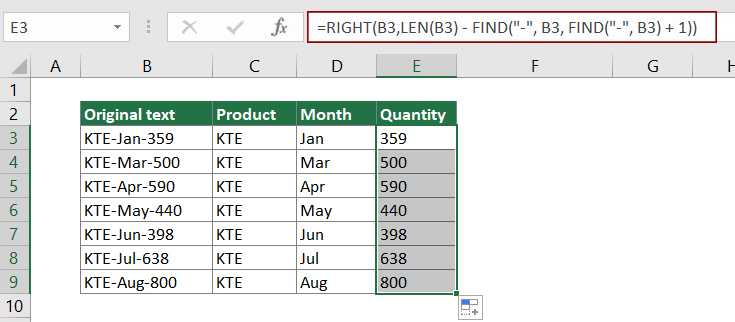Now text string in each cell of column B have been split into three parts.

Tips: If you only want to split the text string into two parts based on a specific character, after splitting the first part with the above LEFT formula, you can apply the below RIGHT formula to extract the second part as the below screenshot shown.

=RIGHT(B3,LEN(B3)-FIND("-",B3))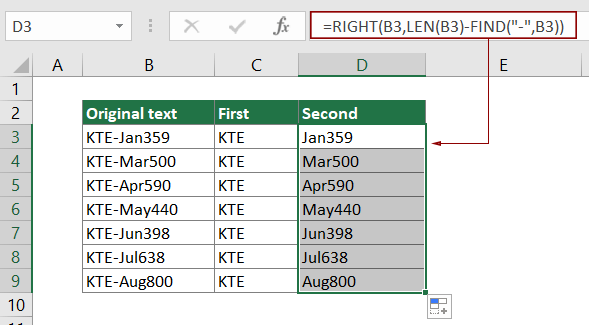How these formulas work?

=LEFT(B3,FIND("-",B3)-1)

• FIND("-",B3): The FIND function returns the starting position of character “-” in the text string KTE-Jan-359. The result is 4.
• LEFT(B3,4-1): The LEFT function extracts 3 characters (4-1=3) from the left side of the text string KTE-Jan-359. Here the result is KTE.
Note: As the first character “-” is in the 4th position of the text string, you need to subtract 1 to exclude it from extracting.

=MID(B3, FIND("-",B3) + 1, FIND("-",B3,FIND("-",B3)+1) - FIND("-",B3) - 1)

• FIND("-",B3) + 1: The FIND function returns the starting position of character “-” in the text string KTE-Jan-359. Here the result is 4.
Note: As the first character "-" is in the 4th position of the text string, you need to add 1 to 4 in order to extract texts after it, and finally we end of with 4+1=5.
• FIND("-",B3,FIND("-",B3)+1) here is shown as FIND("-",KTE-Jan-359,4+1): The Find function finds the position of character "-" starting from the 5th character in KTE-Jan-359. And returns the result as 8.
• MID(B3, 5, 8 - 4 - 1): MID(KTE-Jan-359,5,3) which means that the MID function extracts 3 number of characters starting from the 5th character in the text string KTE-Jan-359. And the result is Jan.

=RIGHT(B3,LEN(B3) - FIND("-", B3, FIND("-", B3) + 1))

• LEN(B3): The LEN function calculates the total length of text string KTE-Jan-359, and returns the result as 11.
• FIND("-",B3,FIND("-",B3)+1): The Find function finds the position of character "-" starting from the 5th character in KTE-Jan-359. And returns the result as 8.
• RIGHT(B3,11 - 8)): The RIGHT function extracts 3 (11-8) characters from the right side of KTE-Jan-359. Here the result is 359.

#### Related functions

Excel LEFT function
The LEFT function extracts the given number of characters from the left side of a supplied string.

Excel FIND function
The FIND function is used to find a string within another string, and returns the starting position of the string inside another one.

Excel MID function
The MID function is used to find and return a specific number of characters from the middle of given text string.

Excel RIGHT function
The RIGHT function extracts a specific number of characters from the right side of the text string.

Excel LEN function
The Excel LEN function returns the number of characters in a text string.

#### Related formulas

Split dimensions into two parts in Excel
This article explains how to use formulas to split dimensions in a cell into two parts without units (individual length and width).

Separate numbers from units of measurement
This article explains how to use formula to separate numbers from unites of measurement.

Split text and numbers in a cell in Excel
If you want to split text and numbers in a cell into different column cells with formulas, this tutorial will do you a favor.

Split Text With Delimiter In A Cell In Excel

### The Best Office Productivity Tools

#### Kutools for Excel - Helps You To Stand Out From Crowd

 Popular Features: Find, Highlight or Identify Duplicates  |  Delete Blank Rows  |  Combine Columns or Cells without Losing Data  |  Round without Formula ... Super VLookup: Multiple Criteria  |  Multiple Value  |  Across Multi-Sheets  |  Fuzzy Lookup... Adv. Drop-down List: Easy Drop Down List  |  Dependent Drop Down List  |  Multi-select Drop Down List... Column Manager: Add a Specific Number of Columns  |  Move Columns  |  Toggle Visibility Status of Hidden Columns  |  Compare Columns to Select Same & Different Cells ... Featured Features: Grid Focus  |  Design View  |  Big Formula Bar  |  Workbook & Sheet Manager | Resource Library (Auto Text)  |  Date Picker  |  Combine Worksheets  |  Encrypt/Decrypt Cells  |  Send Emails by List  |  Super Filter  |  Special Filter (filter bold/italic/strikethrough...) ... Top 15 Toolsets:  12 Text Tools (Add Text, Remove Characters ...)  |  50+ Chart Types (Gantt Chart ...)  |  40+ Practical Formulas (Calculate age based on birthday ...)  |  19 Insertion Tools (Insert QR Code, Insert Picture from Path ...)  |  12 Conversion Tools (Numbers to Words, Currency Conversion ...)  |  7 Merge & Split Tools (Advanced Combine Rows, Split Excel Cells ...)  |  ... and more

Kutools for Excel Boasts Over 300 Features, Ensuring That What You Need is Just A Click Away...#### Office Tab - Enable Tabbed Reading and Editing in Microsoft Office (include Excel)

• One second to switch between dozens of open documents!
• Reduce hundreds of mouse clicks for you every day, say goodbye to mouse hand.
• Increases your productivity by 50% when viewing and editing multiple documents.
• Brings Efficient Tabs to Office (include Excel), Just Like Chrome, Edge and Firefox.